Updating search results...

# 11 Results

View
Selected filters:
• UT.MATH.I.G.CO.3Unrestricted Use
CC BY
Rating
0.0 stars

This is a task from the Illustrative Mathematics website that is one part of a complete illustration of the standard to which it is aligned. Each task has at least one solution and some commentary that addresses important asects of the task and its potential use. Here are the first few lines of the commentary for this task: Lisa makes an octagon by successively folding a square piece of paper as follows. First, she folds the square in half vertically and horizontally and a...

Subject:
Mathematics
Secondary Mathematics
Material Type:
Activity/Lab
Provider:
Illustrative Mathematics
Provider Set:
Illustrative Mathematics
Author:
Illustrative Mathematics
10/14/2013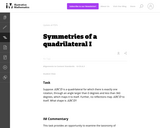Unrestricted Use
CC BY
Rating
0.0 stars

This is a task from the Illustrative Mathematics website that is one part of a complete illustration of the standard to which it is aligned. Each task has at least one solution and some commentary that addresses important asects of the task and its potential use. Here are the first few lines of the commentary for this task: Suppose \$ABCD\$ is a quadrilateral for which there is exactly one rotation, through an angle larger than 0 degrees and less than 360 degrees, which maps...

Subject:
Mathematics
Secondary Mathematics
Material Type:
Activity/Lab
Provider:
Illustrative Mathematics
Provider Set:
Illustrative Mathematics
Author:
Illustrative Mathematics
05/24/2013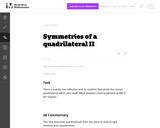Unrestricted Use
CC BY
Rating
0.0 stars

This is a task from the Illustrative Mathematics website that is one part of a complete illustration of the standard to which it is aligned. Each task has at least one solution and some commentary that addresses important asects of the task and its potential use. Here are the first few lines of the commentary for this task: There is exactly one reflection and no rotation that sends the convex quadrilateral ABCD onto itself. What shape(s) could quadrilateral ABCD be? Explai...

Subject:
Mathematics
Secondary Mathematics
Material Type:
Activity/Lab
Provider:
Illustrative Mathematics
Provider Set:
Illustrative Mathematics
Author:
Illustrative Mathematics
05/29/2013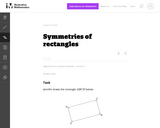Unrestricted Use
CC BY
Rating
0.0 stars

This is a task from the Illustrative Mathematics website that is one part of a complete illustration of the standard to which it is aligned. Each task has at least one solution and some commentary that addresses important asects of the task and its potential use. Here are the first few lines of the commentary for this task: Jennifer draws the rectangle \$ABCD\$ below: Find all rotations and reflections that carry rectangle \$ABCD\$ onto itself. Lisa draws a different rectangle...

Subject:
Mathematics
Secondary Mathematics
Material Type:
Activity/Lab
Provider:
Illustrative Mathematics
Provider Set:
Illustrative Mathematics
Author:
Illustrative Mathematics
05/24/2013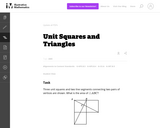Unrestricted Use
CC BY
Rating
0.0 stars

This is a task from the Illustrative Mathematics website that is one part of a complete illustration of the standard to which it is aligned. Each task has at least one solution and some commentary that addresses important asects of the task and its potential use. Here are the first few lines of the commentary for this task: Three unit squares and two line segments connecting two pairs of vertices are shown. What is the area of \$\triangle ABC\$?...

Subject:
Mathematics
Secondary Mathematics
Material Type:
Activity/Lab
Provider:
Illustrative Mathematics
Provider Set:
Illustrative Mathematics
Author:
Illustrative Mathematics
03/27/2013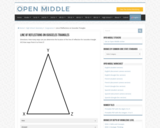Restricted Use
Rating
0.0 stars

Open Middle provides math problems that have a closed beginning, a closed end, and an open middle. This means that there are multiple ways to approach and ultimately solve the problems. Open middle problems generally require a higher Depth of Knowledge than most problems that assess procedural and conceptual understanding.

Subject:
Mathematics
Material Type:
Activity/Lab
Provider:
Open Middle Math
04/26/2022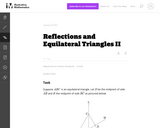Unrestricted Use
CC BY
Rating
0.0 stars

This task examines some of the properties of reflections of the plane which preserve an equilateral triangle: these were introduced in ''Reflections and Isosceles Triangles'' and ''Reflection and Equilateral Triangles I''. The task gives students a chance to see the impact of these reflections on an explicit object and to see that the reflections do not always commute.

Subject:
Mathematics
Secondary Mathematics
Material Type:
Activity/Lab
Provider:
Illustrative Mathematics
Provider Set:
Illustrative Mathematics
Author:
Illustrative Mathematics
01/05/2013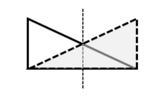Conditional Remix & Share Permitted
CC BY-NC
Rating
0.0 stars

This is a lesson plan that introduces rotational and reflectional symmetry with geometric shapes, focusing on regular polygons. Notes (two copies: a student version and also an answer key, both as a Word doc and a PDF), assignment, and cut-out shapes are provided.

Subject:
Secondary Mathematics
Material Type:
Homework/Assignment
Lesson
Author:
Kimberly
02/07/2022Conditional Remix & Share Permitted
CC BY-NC
Rating
0.0 stars

This is a lesson plan that introduces rotational and reflectional symmetry with geometric shapes, focusing on regular polygons. Notes (two copies: a student version and also an answer key, both as a Word doc and a PDF), assignment, and cut-out shapes are provided. There is a final project, with both instructions and an example included. Cover image: Christensen, K., 2022. Reflectional Symmetry of an Atom [screenshot] (Keynote presentation, Rotational and Reflectional Symmetry Assignment).

Subject:
Secondary Mathematics
Material Type:
Activity/Lab
Assessment
Diagram/Illustration
Homework/Assignment
Lesson Plan
Author:
Kimberly
03/09/2022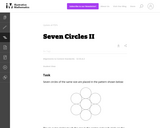Unrestricted Use
CC BY
Rating
0.0 stars

This task provides a concrete geometric setting in which to study rigid transformations of the plane. It is important for students to be able to visualize and execute these transformations and for this purpose it would be beneficial to have manipulatives and it will important that the students be able to label the vertices of the hexagon with which they are working.

Subject:
Mathematics
Secondary Mathematics
Material Type:
Activity/Lab
Provider:
Illustrative Mathematics
Provider Set:
Illustrative Mathematics
Author:
Illustrative Mathematics
08/20/2012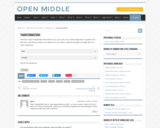Restricted Use